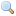# Module type Map.S

`module type S = `sig` .. `end``
Output signature of the functor `Map.Make`.

`type key `
The type of the map keys.
`type `+'a` t `
The type of maps from type `key` to type `'a`.
`val empty : `'a t``
The empty map.
`val is_empty : `'a t -> bool``
Test whether a map is empty or not.
`val mem : `key -> 'a t -> bool``
`mem x m` returns `true` if `m` contains a binding for `x`, and `false` otherwise.
`val add : `key -> 'a -> 'a t -> 'a t``
`add x y m` returns a map containing the same bindings as `m`, plus a binding of `x` to `y`. If `x` was already bound in `m`, its previous binding disappears.
`val singleton : `key -> 'a -> 'a t``
`singleton x y` returns the one-element map that contains a binding `y` for `x`.
Since 3.12.0
`val remove : `key -> 'a t -> 'a t``
`remove x m` returns a map containing the same bindings as `m`, except for `x` which is unbound in the returned map.
`val merge : `(key -> 'a option -> 'b option -> 'c option) ->       'a t -> 'b t -> 'c t``
`merge f m1 m2` computes a map whose keys is a subset of keys of `m1` and of `m2`. The presence of each such binding, and the corresponding value, is determined with the function `f`.
Since 3.12.0
`val compare : `('a -> 'a -> int) -> 'a t -> 'a t -> int``
Total ordering between maps. The first argument is a total ordering used to compare data associated with equal keys in the two maps.
`val equal : `('a -> 'a -> bool) -> 'a t -> 'a t -> bool``
`equal cmp m1 m2` tests whether the maps `m1` and `m2` are equal, that is, contain equal keys and associate them with equal data. `cmp` is the equality predicate used to compare the data associated with the keys.
`val iter : `(key -> 'a -> unit) -> 'a t -> unit``
`iter f m` applies `f` to all bindings in map `m`. `f` receives the key as first argument, and the associated value as second argument. The bindings are passed to `f` in increasing order with respect to the ordering over the type of the keys.
`val fold : `(key -> 'a -> 'b -> 'b) -> 'a t -> 'b -> 'b``
`fold f m a` computes `(f kN dN ... (f k1 d1 a)...)`, where `k1 ... kN` are the keys of all bindings in `m` (in increasing order), and `d1 ... dN` are the associated data.
`val for_all : `(key -> 'a -> bool) -> 'a t -> bool``
`for_all p m` checks if all the bindings of the map satisfy the predicate `p`.
Since 3.12.0
`val exists : `(key -> 'a -> bool) -> 'a t -> bool``
`exists p m` checks if at least one binding of the map satisfy the predicate `p`.
Since 3.12.0
`val filter : `(key -> 'a -> bool) -> 'a t -> 'a t``
`filter p m` returns the map with all the bindings in `m` that satisfy predicate `p`.
Since 3.12.0
`val partition : `(key -> 'a -> bool) -> 'a t -> 'a t * 'a t``
`partition p m` returns a pair of maps `(m1, m2)`, where `m1` contains all the bindings of `s` that satisfy the predicate `p`, and `m2` is the map with all the bindings of `s` that do not satisfy `p`.
Since 3.12.0
`val cardinal : `'a t -> int``
Return the number of bindings of a map.
Since 3.12.0
`val bindings : `'a t -> (key * 'a) list``
Return the list of all bindings of the given map. The returned list is sorted in increasing order with respect to the ordering `Ord.compare`, where `Ord` is the argument given to `Map.Make`.
Since 3.12.0
`val min_binding : `'a t -> key * 'a``
Return the smallest binding of the given map (with respect to the `Ord.compare` ordering), or raise `Not_found` if the map is empty.
Since 3.12.0
`val max_binding : `'a t -> key * 'a``
Same as `Map.S.min_binding`, but returns the largest binding of the given map.
Since 3.12.0
`val choose : `'a t -> key * 'a``
Return one binding of the given map, or raise `Not_found` if the map is empty. Which binding is chosen is unspecified, but equal bindings will be chosen for equal maps.
Since 3.12.0
`val split : `key -> 'a t -> 'a t * 'a option * 'a t``
`split x m` returns a triple `(l, data, r)`, where `l` is the map with all the bindings of `m` whose key is strictly less than `x`; `r` is the map with all the bindings of `m` whose key is strictly greater than `x`; `data` is `None` if `m` contains no binding for `x`, or `Some v` if `m` binds `v` to `x`.
Since 3.12.0
`val find : `key -> 'a t -> 'a``
`find x m` returns the current binding of `x` in `m`, or raises `Not_found` if no such binding exists.
`val map : `('a -> 'b) -> 'a t -> 'b t``
`map f m` returns a map with same domain as `m`, where the associated value `a` of all bindings of `m` has been replaced by the result of the application of `f` to `a`. The bindings are passed to `f` in increasing order with respect to the ordering over the type of the keys.
`val mapi : `(key -> 'a -> 'b) -> 'a t -> 'b t``
Same as `Map.S.map`, but the function receives as arguments both the key and the associated value for each binding of the map.